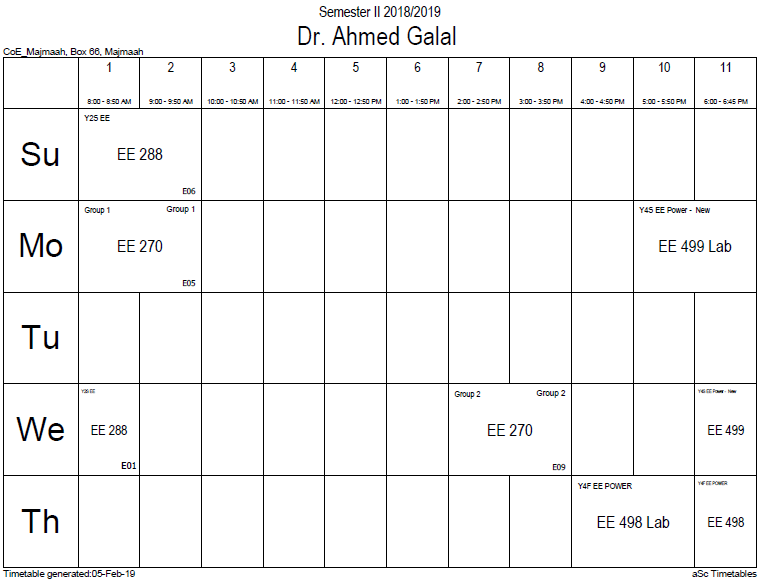## Wave functions and probabilities

If the configuration space X is continuous (something like the real line or Euclidean space, see above), then there are no valid quantum states corresponding to particular xX, and the probability that the system is "in the state x" will always be zero. An archetypical example of this is the L2(R) space constructed with 1-dimensional Lebesgue measure; it is used to study a motion in one dimension. This presentation of the infinite-dimensional Hilbert space corresponds to the spectral decomposition of the coordinate operator: x | Q | Ψ = xx | Ψ, xR in this example. Although there are no such vectors as x |, strictly speaking, the expression x | Ψ can be made meaningful, for instance, with spectral theory.

Generally, it is the case when the motion of a particle is described in the position space, where the corresponding probability amplitude function ψ is the wave function.

If the function ψL2(X), ‖ψ‖ = 1 represents the quantum state vector , then the real expression |ψ(x)|2, that depends on x, forms a probability density function of the given state. The difference of a density function from simply a numerical probability means that one should integrate this modulus-squared function over some (small) domains in X to obtain probability values – as was stated above, the system can't be in some state x with a positive probability. It gives to both amplitude and density function a physical dimension, unlike a dimensionless probability. For example, for a 3-dimensional wave function the amplitude has a "bizarre" dimension [L−3/2].

Note that for both continuous and infinite discrete cases not every measurable, or even smooth function (i.e. a possible wave function) defines an element of L2(X); see #Normalisation below.

### Office HoursNo office hours### My Timetable### Contactsemail: [email protected]

Phone: 2570

### Welcome

Welcome To Faculty of Engineering### IEEEhttp://www.ieee.org/

/

### Bookmarkshttp://www.utk.edu/research/

http://science.doe.gov/grants/index.asp

http://www1.eere.energy.gov/vehiclesandfuels/

http://www.eere.energy.gov/

### Upcoming Conferences### Engineering quotes### Travel Web Siteshttp://www.hotels.com/

http://www.orbitz.com/

http://www.hotwire.com/us/index.jsp

http://www.kayak.com/

### Blackboardستقام اختبارات الميدتيرم يوم الثلاثاء 26-6-1440

حسب الجدول المعلن بلوحات الاعلان

### Summer trainingThe registration for summer training will start from 5th week of second semesterClass registration week 1

### برنامج التجسير### إحصائية الموقع

عدد الصفحات: 2879

البحوث والمحاضرات: 1280

الزيارات: 100969